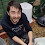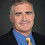## Tuesday, April 05, 2011

### A New Proof of the Nondeterministic Time Hierarchy

A nondeterministic time hierarchy was first proved by Cook and in the strongest form by Seiferas, Fischer and Meyer.  Zàk gave a simple proof that we sketched in this post. Here is another.

Theorem: If t1 and t2 are time-constructible functions and t1(n+1)=o(t2(n)) then NTIME(t1(n)) is strictly contained in NTIME(t2(n)).

Proof:

Let M1,… be an enumeration of nondeterministic Turing machines. We define a nondeterministic machine M that acts as follows on input w=1i01m0y
• If |y|<t1(i+m+2) then accept if Mi accepts both inputs 1i01m0y0 and 1i01m0y1 in t2(|w|) steps.
• If |y|=t1(i+m+2) then accept if Mrejects input 1i01m0 on the computation path described by y.
This machine uses time O(t2(n)). If NTIME(t1(n))=NTIME(t2(n)) then there is an equivalent machine Mi using time O(t1(n)).
Since t1(n+1)=o(t2(n)) we have for sufficiently large m,

1i01m0 in L(M) ⇔ 1i01m0y in L(M) for |y|=1⇔ … ⇔ 1i01m01y in L(M) for |y|=t1(i+m+2)⇔ M(1i01m0) rejects on all computation paths y

The advantage over Zàk is that you only need t1 steps instead of exponential in t1. On the other hand Zàk can give you a unary language and can be generalized to a broader set of complexity measures.

Rahul Santhanam and I needed and discovered this proof for our recent paper. The proof came out of a failed attempt at an oracle to show that no such relativized proof would be possible.

1.I believe that t_2(|w|) in the line of the first bullet is t_1(|w|).

Bin Fu

2.Bin Fu: I'm not sure it would make any difference. Maybe the error there is that "|y|=t_1(m)" should read "|y| = t_1(m+i+2)" ?

3.Now I feel Lance's proof is fine.
It does not need any revision.
It is a very nice proof for this
important theorem.

Bin

4.Hi Lance,
Could you explain why does the machine M run in non-deterministic time t_2?

I am probably missing something, but don't you need need time of at least 2^t_1(n) in order to emulate M_i on all possible computation paths?

Thanks!
Or

5.What "broader set of complexity measures" are you referring to?

6.The proof is amazingly compact. It's only 10 lines long, which gives it elegance, sort of a Sudoku square stripped to its essential clues from which the full solution can be expanded. I'm stuck (I didn't get today's Sudoku either though), here's where. The proof defines a machine M. There are two clauses in the definition: M accepts if condition 1, and M accepts if condition 2. So I provisionally believe that M is a machine which can never reject anything, as that's not in its nature. But later in the proof there's a clause "M rejects", at least I think it's M and not some other indexed nondeterministic Turing machine, which threatens my provisional belief. Back to square 1 !

7.OK, I see it now. Thanks to Arnab Bhattacharyya for a patient explanation.

Very nice proof!

8.Michaël: Good catch. Fixed.

Or: In the second bullet, M only simulates M_i on the single path described by y.

Anon 5: Zàk's proof automatically works for any measure that has universal simulation (like Sigma_5-Time). It's much harder to generalize our techniques.

9.Brilliant proof!

10.Btw, I really wonder if my previous comment goes through moderation - on one hand, it is not offensive at all, on the other, it does not add much, so it might seem immodest if you let it appear. Meta: Will this comment go through?

11.We publish all comments good and bad. We moderate to stop the ugly.

12.I'm trying to give this proof in class, but am missing something. Consider the reverse direction. Say $1^i 0 1^m 0 \not\in L(M)$. Then we know that M_i rejects on all computation path. But M_i runs in time O(t_1(n)), not t_1(n). So maybe all its computation paths have length 2t_1(n), say. (And I don't think you can use the speedup theorem here, without allowing y to be non-binary. Isn't that right?) So then M_i rejects on computation path y for all y of length 2t_1(n). But then the argument stops.

What am I missing?

13.I guess machine M actually needs to check if |y|=t_2(i+m+2). That seems to work. (Note: the error, if it is one, appears to be in the paper as well.)

14.Dear Lance, it looks like the proof also works for deterministic machines. So, we should have DTIME(t1(n)) strictly contained in DTIME(t2(n)) as soon as t1(n+1) = o(t2(n)) and both t1, t2 time constructible. But then where is the log factor that usually appears in deterministic time hierarchy?

--Martin & Stephan from Munich

1.For deterministic computation you need an extra log factor for the simulation, technically a log factor to reduce from k-tapes to 2-tapes. For nondeterministic there is a way to get from k-tapes to 2-tapes without an extra log overhead.

15.Nice! It solves an open problem posed in our book, asking whether the exponential "gap" in the standard proof is inherent.